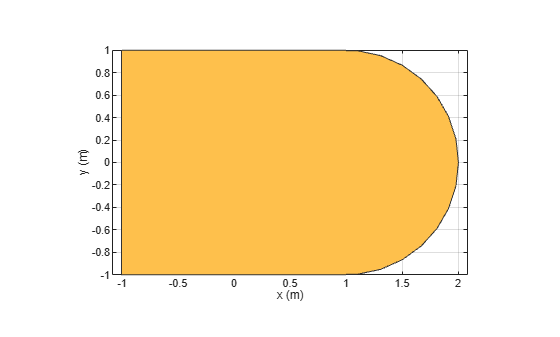Boolean unite operation on two shapes

## Syntax

``c = add(shape1,shape2)``

## Description

example

````c = add(shape1,shape2)` unites `shape1` and `shape2` using the add operation. You can also use the `+` to add the two shapes together.```

## Examples

collapse all

Create and view a default circle.

`circle1 = antenna.Circle;`

Create a circle with a radius of 1 m. The center of the circle is at [1 0].

`circle2 = antenna.Circle('Center',[1 0],'Radius',1);`

`add(circle1,circle2)`Create a circle with a radius of 1m. The center of the circle is at [1 0].

`circle1 = antenna.Circle(Center=[1 0],Radius=1);`

Create a rectangle with a length of 2m and a width of 4m centered at the origin.

`rect1 = antenna.Rectangle(Length=2,Width=2);`

Add the two shapes together using the `+` function.

`polygon1 = circle1+rect1`
```polygon1 = Polygon with properties: Name: 'mypolygon' Vertices: [21x3 double] ```
`show(polygon1)`## Input Arguments

collapse all

Shapes created using custom elements and shape objects of Antenna Toolbox™, specified as an object.

Example: `c = add(rectangle1, rectangle2)`, where rectangle1 and rectangle2 are shapes created using `antenna.Rectangle` object.

## Version History

Introduced in R2017a